# MP Board Class 11th Maths Important Questions Chapter 8 Binomial Theorem

## MP Board Class 11th Maths Important Questions Chapter 8 Binomial Theorem

### Binomial Theorem Important Questions

Binomial Theorem Objective Type Questions

(A) Choose the correct option :

Question 1.
The total number of terms in the expansion of
(a) 7
(b) 12
(c) 13
(d) 6.
(c) 13

Question 2.
If y = 3x + 6x2 + 10x3 + …………… ∞, then the correct relation will be :
(a) x = 1 – (1 + y)–$$\frac { 1 }{ 3 }$$
(b) x = (1 + y)–$$\frac { 1 }{ 3 }$$
(c) y = 1 – (1 – x)-3
(d) x = 1 + (1 + y)–$$\frac { 1 }{ 3 }$$
(a) x = 1 – (1 + y)–$$\frac { 1 }{ 3 }$$

Question 3.
The total number of terms in the expansion of (1+ x)-1 will be :
(a) 0
(b) ∞
(c) 2
(d) It can not be expand
(b) ∞Question 4.
The mid – term in the expansion of (x – $$\frac { 1 }{ x }$$)10 will be :
(a) – 10C5
(b) 10C5
(c) 251
(d) 252
(a) – 10C5

Question 5.
For all positive integer of n, n(n – 1) is :
(a) Integer
(b) Natural number
(c) Even positive integer
(d) Odd positive integer.
(c) Even positive integer

Question 6.
Expansion of (a + x)n is :
(a) an + nC1an-1x + nC2an-2x2 + ……….. + nCran-rxr + ………… + an
(b) xn + nC1xn-1a +nC2xn-2a2 + ……….. + nCrxn-rar + ………… + an
(c) annC1an-1x + nC2an-2x2 + ……….. + (-1)rnCran-rxr + ………… + (-1)nan
(d) xnnC1an-1a + nC2an-2a2 + ……….. + (-1)rnCran-rxr + ………… + (-1)nxn
(a) an + nC1an-1x +nC2an-2x2 + ……….. + nCran-rxr + ………… + an

Question 7.
The fifth term in the expansion of ( x – $$\frac { 1 }{ x }$$ )10 from the end, will be :
(a) $$\frac { ^{ 10 }{ C }_{ 6 } }{ x }$$
(b) $$\frac { 105 }{ 32{ x }^{ 2 } }$$
(c) $$\frac { ^{ 10 }{ C }_{ 6 } }{ { x }^{ 2 } }$$
(d) $$\frac { ^{ 10 }{ C }_{ 6 } }{ { x }^{ 10 } }$$
(a) $$\frac { ^{ 10 }{ C }_{ 6 } }{ x }$$
[Hint: The total number of terms be 11 in the expansion of it.
∵ The 5th term from end = (11 – 5)th = 7th term from the beginning.]

Question 8.
The number of mid – terms in the expansion of ( x – $$\frac { 1 }{ x }$$ )10
(a) 1
(b) 2
(c) – $$\frac { ^{ 13 }{ C }_{ 7 } }{ x }$$
(d) 1716x
(b) 2

Question 9.
The value of nC0 + nC1 + nC2 + …………….. + nCn :
(a) 2n + 1
(b) 2n – 1
(c) 2n – 1
(d) 2n
(d) 2n

Question 10.
The value of nC0 + nC2 + nC4 + …………….. = nC1 + nC3 + ……………. will be :
(a) 2n + 1
(b) 2n – 1
(c) 2n – 1
(d) 2n
(b) 2n – 1

Question 11.
The total number of terms in the expansion of (a + b + c + d)n will be :
(a) $$\frac { (n+1)(n+2) }{ 2 }$$
(b) $$\frac { n(n+1) }{ 2 }$$
(c) $$\frac { (n+1)(n+2)(n+3) }{ 6 }$$
(d) $$\frac { (n+1)(n+2) }{ 6 }$$
(c) $$\frac { (n+1)(n+2)(n+3) }{ 6 }$$

Question 12.
The necessary condition for expansion of (1 + x)-1 is :
(a) | x | < 1
(b) | x | > 1
(c) | x | = 1
(d) | x | = – 1.
(a) | x | < 1

Question 13.
The general term in the expansion of (x + a)n will be :
(a) rth
(b) (r+1)thterm
(c) (r-1)th
(d) (r+2)thterm
(b) (r+1)thterm

Question 14.
In the expansion of ( 2x + $$\frac { 1 }{ { 3x }^{ 2 } }$$ )9, then term independent of x will be :
(a) $$\frac { 8 }{ 127 }$$
(b) $$\frac { 124 }{ 81 }$$
(c) $$\frac { 1792 }{ 9 }$$
(d) $$\frac { 256 }{ 243 }$$
(c) $$\frac { 1792 }{ 9 }$$

Question 15.
The coefficient of x3 in the expansion of ( x – $$\frac { 1 }{ x }$$ )15 is :
(a) 14
(b) 21
(c) 28
(d) 35
(b) 21

Question 16.
The independent term in the expansion of (x2 – $$\frac { 2 }{ { x }^{ 3 } }$$)15 is :
(a) 5th
(b) 6th
(c) 7th
(d) 8th
(c) 7thQuestion 17.
The value of nC0 + nC1 + nC2 + …………….. = nCn the expansion of (l + x)n is :
(a) 2n – 1
(b) 2n – 2
(c) 2n
(d) 2n-1
(c) 2n

Question 18.
The value of 15C0 + 15C2 + 15C4 + 15C6 + …………….. = 15C14 is :
(a) 214
(b) 215
(c) 215 – 1
(d) None of these
(a) 214

(B) Match the following :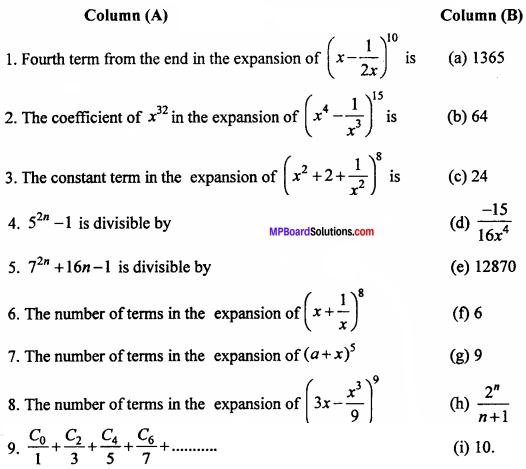1. (d)
2. (a)
3. (e)
4. (c)
5. (b)
6. (g)
7. (f)
8. (i)
9. (b)

(C) Fill in the blanks :

1. By binomial theorem the value of (102)4 is ……………..
2. The value of second term in the expansion of (1 – x)-3/2 is ……………..
3. The 5th term from the end in the expansion of (x – $$\frac { 1 }{ 2x }$$ )10 is ……………..
4. The constant term will be …………….. in the expansion of ( x2 – 2 + $$\frac { 1 }{ { x }^{ 2 } }$$ )6
5. The value of C1 + 2C2 + 3C3 + ………….. + nCn will be ……………..
6. The value of nC0nC1 + nC2nC3 + ………….. will be ……………..
7. The value of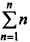is ……………..
8. The value of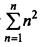is ……………..
9. The value of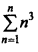is ……………..
10. (2x + 3y)5 = …………….. up to three terms.
11. The coefficient of x7 in the expansion of (x2 + $$\frac { 1 }{ x }$$ )11 will be …………….
12. In the expansion of (1 – x)10 the value of middle term is ……………..
13. Third (3rd) term in the expansion of e-3x will be ……………..
14. If n is odd, in the expansion of (x + a)n, then number of middle terms are ……………..
15. The middle term in the expansion of ($$\frac { x }{ a }$$ + $$\frac { a }{ x }$$ )10
16. The coefficient of xn in the expansion of (1 + x) (1 – x)n will be ……………..

1. 08243216
2. $$\frac { { x }^{ 3 } }{ 16 }$$
3. $$\frac { 105 }{ 32{ x }^{ 2 } }$$
4. 924
5. n.2n-1
6. 0
7. $$\frac { n(n+1) }{ 2 }$$
8. $$\frac { n(n+1)(2n+1) }{ 6 }$$
9. [ $$\frac { n(n+1) }{ 2 }$$ ]2
10. 32x5 + 240x4y + 720x3y2
11. 462
12. – 252 x-5
13. $$\frac { 1 }{ 2 }$$
14. Two
15. 252
16. (- 1)n(1 – n)

(D) Write true / false :

1. The expansion of (1 + x)-3 is 1 – 3x + 6x2 – 10x3 + ………….. + $$\frac { (- 1)(r + 1)(r +2) }{ 2! }$$xr + ……………..
2. The expansion of (1 – x)-3 is 1 + 3x + 6x2 + 10x3 + ………….. + $$\frac { (- 1)r(r + 1)(r +2) }{ 2! }$$xr + ……………..
3. The expansion of (1 – x)-2 is 1 + 2x + 3x2 + (r + 1) xr+ ………….. +
4. The (r + 1 )th term in the expansion of (1 – x)-2 is (- 1)r(r + 1) xr+ ………….. +
5. The (r + 1 )th term in the expansion of (1 – x)n will be xr
6. The total number of terms in the expansion of (a + b + c)n is $$\frac { (n + 1)(n + 1) }{ 2 }$$
7. In the expansion of ( 3x – $$\frac { { x }^{ 3 } }{ 9 }$$ )9, No . of terms is 9.
8. The number of term in the expansion of ( 3x – $$\frac { { x }^{ 3 } }{ 9 }$$ )9 is 8.
9. In the expansion of (x + a)n then sum of powers of x and α in any term is n.
10. The coefficient of x in the expansion of (1 – 2x)-3 is 6.
11. The second term in the expansion of (2x + 3y)5 is 240x4y.
12. The value of second term in the expansion of (1 – x)-3/2 is $$\frac { 3 }{ 2 }$$x.

1. True
2. True
3. True
4. True
5. False
6. True
7. False
8. False
9. True
10. False
11. True
12. True.

(E) Write answer in one word / sentence :

1. Find the value of 9993 by the binomial theorem
2. Find the middle term in expansion of (x2 – $$\frac { 1 }{ x }$$)6
3. General term in the expansion of (x + a)n will be.
4. If in the expansion of (1+x)51 the coefficient of xr and xr – 5 are equal, then the value of r will be.
5. Find the coefficient of xn in the expansion of (1 + x + x2 + …………… ∞)2, if | x | < 1.
6. In the expansion of ($$\frac { x }{ 3 }$$ – $$\frac { 2 }{ { x }^{ 2 } }$$)10, x4 comes in rth term, then the value of r will be.
7. The 5th term in the expansion of (1 – 2x)– 1 will be.

1. 997002999
2. – 20 x5
3. nCr xn – r ar
4. 28
5. (n + 1)
6. 3
7. 16x2

Binomial Theorem Long Answer Type Questions

Question 1.
Expand : ($$\frac { 2 }{ x }$$ – $$\frac { x }{ 2 }$$)5 (NCERT)
Solution: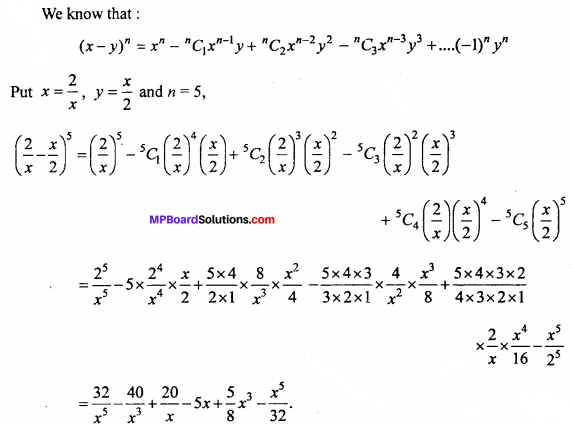Question 2.
Expand : (2x – 3)6 (NCERT)
Solution: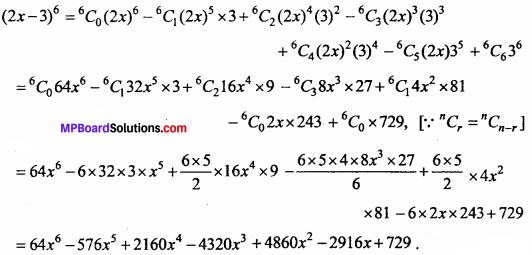Question 3.
Expand : ($$\frac { x }{ 3 }$$ + $$\frac { 1 }{ x }$$)5 (NCERT)
Solution: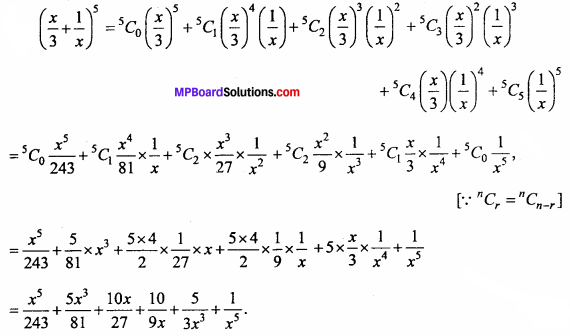Question 4.
Expand : (x + $$\frac { 1 }{ x }$$)6. (NCERT)
Solution: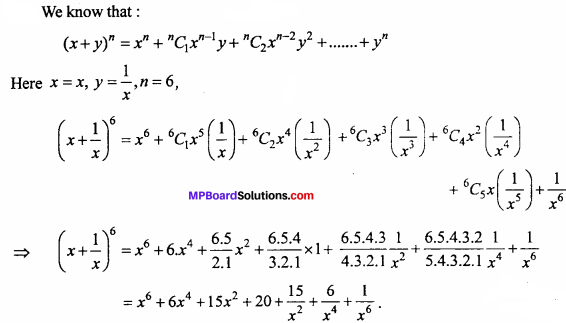Question 5.
find 13th term in the expansion of ( 9x – $$\frac { 1 }{ 3\sqrt { x } }$$ )18. (NCERT)
Solution: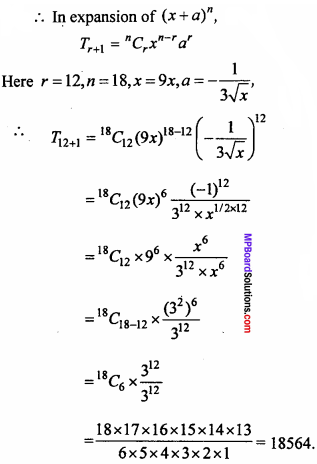Question 6.
Find the middle term of (3 – $$\frac { { x }^{ 3 } }{ 6 }$$)7
Solution: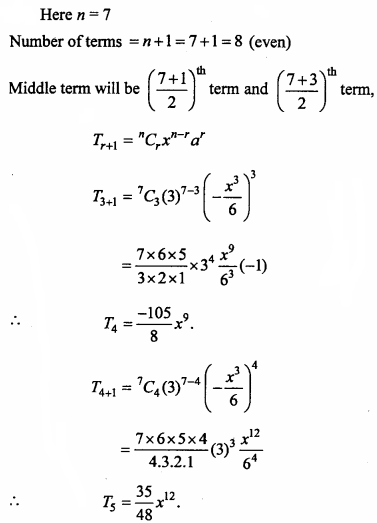Question 7.
Find the middle term in the expansion of ($$\frac { x }{ 3 }$$ + 9y)10
Solution:
Here n =10
Total number of terms = n + 1 = 10 + 1 = 11 (odd)
Here, the term will be middle term.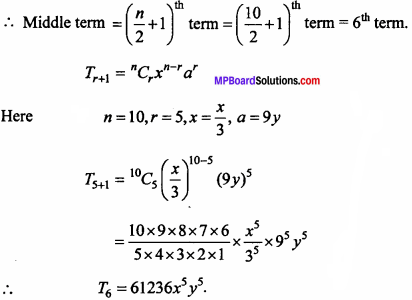Question 8.
If coefficient of x2 and x3 in the expansion of (3 + ax)9 are equal, the value of a.
Solution: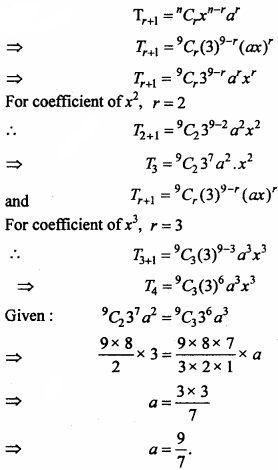Question 9.
Find the coefficient of x5 in the expansion of (x + 3)8
Solution:
Suppose x5 appears in (r + 1)th term Tr+1 = nC1xn-rar
Here n = 8, x = x, a = 3
Tr+1 = 8Cr(x)8 – r(3)r
For the coefficient of x5,
8 – r = 5
=> r = 3
T3+1 = 8C3(3)3
= $$\frac { 8 × 7 × 6 }{ 3 × 2 × 1}$$ × 3 × 3 × 3 × x5
= 1512 × x5
Hence coefficient of x5 is 1512.Question 10.
Find the coefficient of a5b7 in the expansion of (a – 2b)12.
Solution: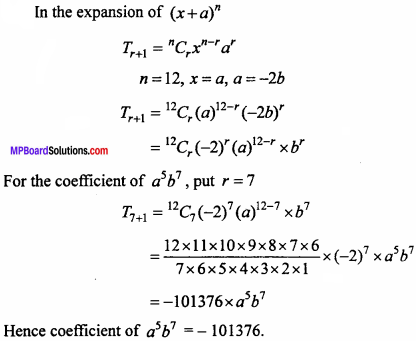Question 11.
If the 17th and 18th terms in the expansion of (2 + a)50 are equal, then find the value of a. (NCERT)
Solution:
In the expansion of (x + a)n
Tr+1 = nCr xn – r ar
Here n = 50, x = 2, a = a
T17 = T16 + 1 = 50C16 (2)50 – 16 (a)16
⇒ T17 = 50C16 (2)34 (a)16
and T18 = T17 + 1 = 50C17 (2)50 – 17 (a)17
= 50C17 (2)33 (a)17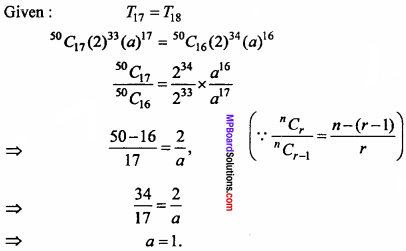Question 12.
Prove that the value of the middle term in the expansion of (1+x)2n is $$\frac { { 1.3.5 …….. (2n – 1)} }{ n! }$$.2n xn
Solution: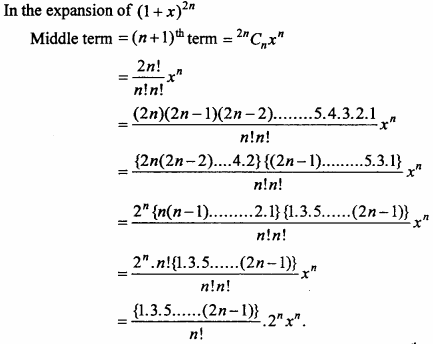Question 13.
In the expansion of (x + 1)n, the coefficient of the (r – 1)th, rth and (r + 1)th terms are in the ratio 1 : 3 : 5, then find the value of n and r.
Solution:
In the expansion of (x + 1)n,
Tr + 1 = nCrxn – r(1)r
Tr – 1 = Tr – 2 + 1 = nCr – 2(x)n – (r – 2)(1)r – 2
Coefficient of Tr – 1th term = nCr – 2
Tr = Tr – 1 + 1 = nCr – 1(x)n – (r – 1)(1)r – 1
Coefficient of Trthterm = nCr – 1
Tr + 1 = nCr xn – r (1)r
Coefficient of Tr+1th term = nCr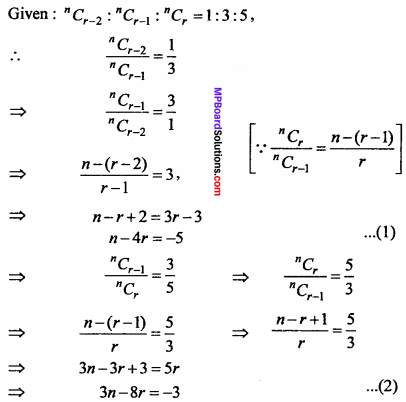Put n = 4r – 5 from equation (1) in equation (2),
3(4r – 5) – 8r = – 3
⇒ 12r – 15 – 8r = – 3
⇒ 4r = 12
∴ r = 3
Put r = 3 in equation (2),
n – 4 x 3 = – 5
⇒ n = 12 – 5
⇒ n = l
n = 7, r = 3

Question 14.
Prove that the coefficient of xn in the expansion of (1 + x)2n of in the expansion of (1 + x)2n – 1.
Solution:
In the expansion of (x + a)n
Tr+1 = nCr xn – r ar
Here x = 1, a = x, n = 2n
Tr+1 = 2nCr(1)2n – r(x)r
For the coefficient of xn, put r = n,
Tn+1 = 2nCn(a)2n – n(x)n
and T18 = T17 + 1 = 50C17 (2)50 – 17 (a)17
= (2nCn) xn
∴ In the expansion of (1 + x)2n, the coefficient of xn = 2nCn …. (1)
and in the expansion of (1 + x)2n – 1, x = 1, a = x, n = 2n – 1
∴ Tr+1 = 2nCr (1)2n – 1  -r (x)n
For the coefficient of xn, put r = n, ‘
We get Tn+1 = 2n – 1Cn xn
The coefficient of xn in the expansion of (1 + x)2n – 1 = n – 1Cn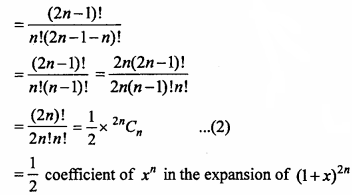∴ The coefficient of xn in the expansion of (1 + x)2n
= 2 x The coefficient of xn in the expansion of (1 + x)2n, [from equation (1) and (2)]

Question 15.
Find the constant term in the expansion of ($$\frac { 3 }{ 2 }$$x2 – $$\frac { 1 }{ 3x }$$)6
Solution: